## Example Questions

← Previous 1

### Example Question #1 : How To Graph A Point

How would you plot the point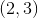?

From the origin, go rightunits, and downunits

From the origin, go rightunits, and upunits

From the origin, go upunits, and rightunits

From the origin, go leftunits, and upunits

From the origin, go rightunits, and upunits

Explanation:

For the ordered pairThe first number is for the x-axis, so because it is positive you go right.

The second number is for the y-axis, so because it is positive you go up.

### Example Question #2 : How To Graph A Point

How would you plot the point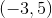?

From the origin, go leftunits, and upunits

From the origin, go leftunits, and downunits

From the origin, go rightunits, and downunits

From the origin, go rightunits, and upunits

From the origin, go leftunits, and upunits

Explanation:

For the ordered pairwe would do the following.

The first number is for the x-axis, so because it is negative you go leftunits.

The second number is for the y-axis, so because it is positive you go upunits.

### Example Question #1 : How To Graph A Point

In which Quadrant is the point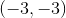located?

Explanation:

To find out which quadrant a point lives in, we need to remember the qualities of each quadrant. By qualities, I mean the signs associated with the x and y values which are dependent on the quadrant the point resides in.

All coordinate pairs that are in Quadrant I will have a positive x value and a positive y value.

All coordinate pairs that are in Quadrant II will have a negative x value and a positive y value.

All coordinate pairs that are in Quadrant III will have a negative x value and a negative y value.

All coordinate pairs that are in Quadrant IV will have a positive x value and a negative y value.

For the pointwe see that both the x and y values are negative therefore, the point lies in the third quadrant. We can also check this by starting from the origin and going left 3 units and down 3 units. This will end up in the lower-left section of the coordinate-plane, which is Quadrant III.

### Example Question #1 : How To Graph A Point

What are the coordinates of point B?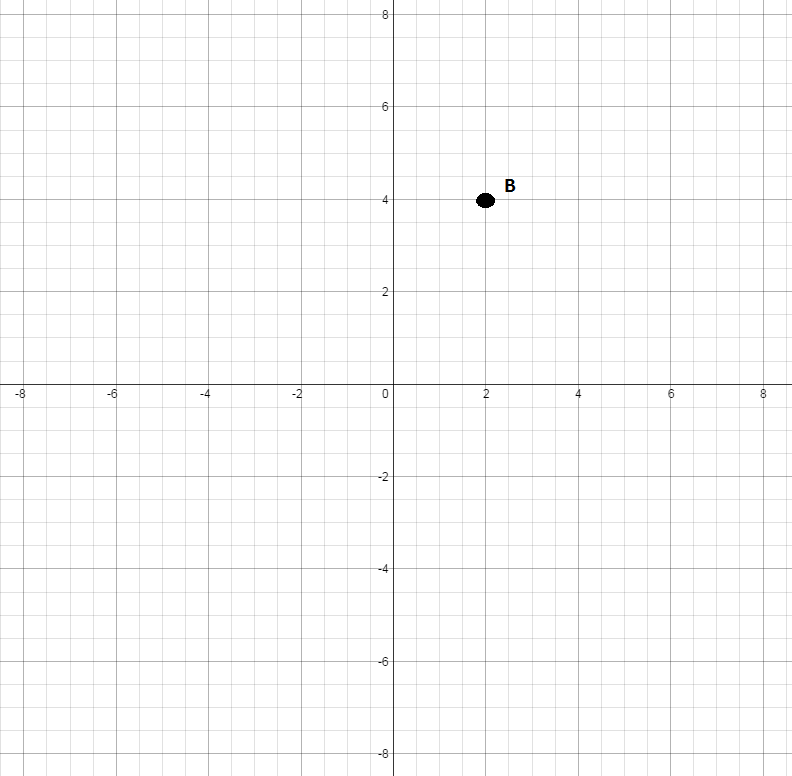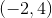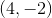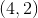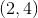Explanation:

Find the x-coordinate by going right on the horizontal x-axis until you come across the line that is directly under the point. The x-coordinate is.

Now, continue up until you reach the point and look across to the vertical y-axis. The y-coordinate is.is then the coordinate of the point.

### Example Question #2 : How To Graph A Point

What are the coordinates for point A?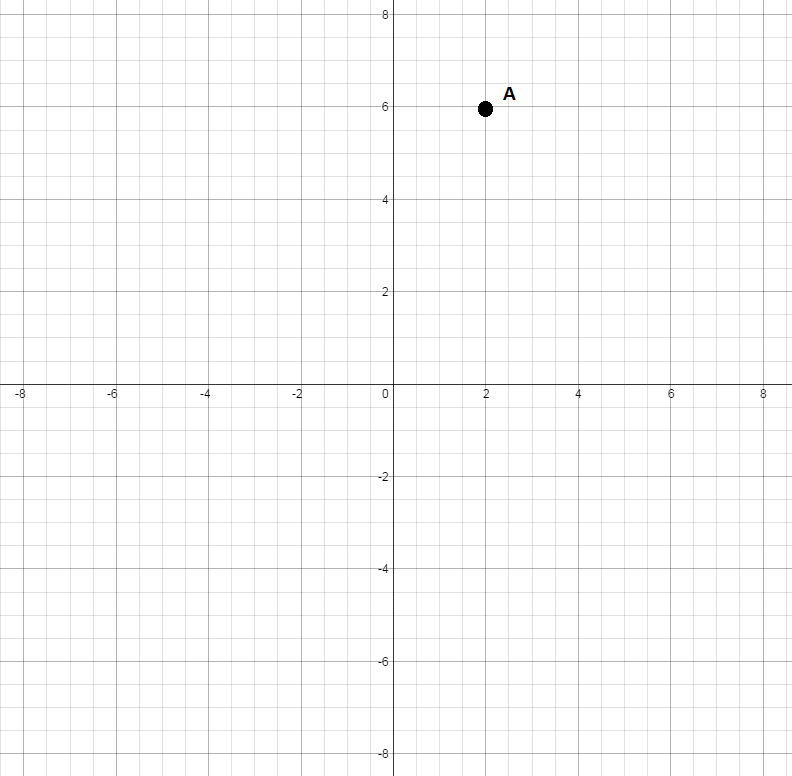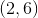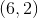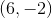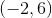Explanation:

Find the x-coordinate by going right on the horizontal x-axis until you come across the line that is directly under the point. The x-coordinate is.

Now, continue up until you reach the point and look across to the vertical y-axis. The y-coordinate is.are the coordinates for point A.

### Example Question #3 : How To Graph A Point

What are the coordinates for point C?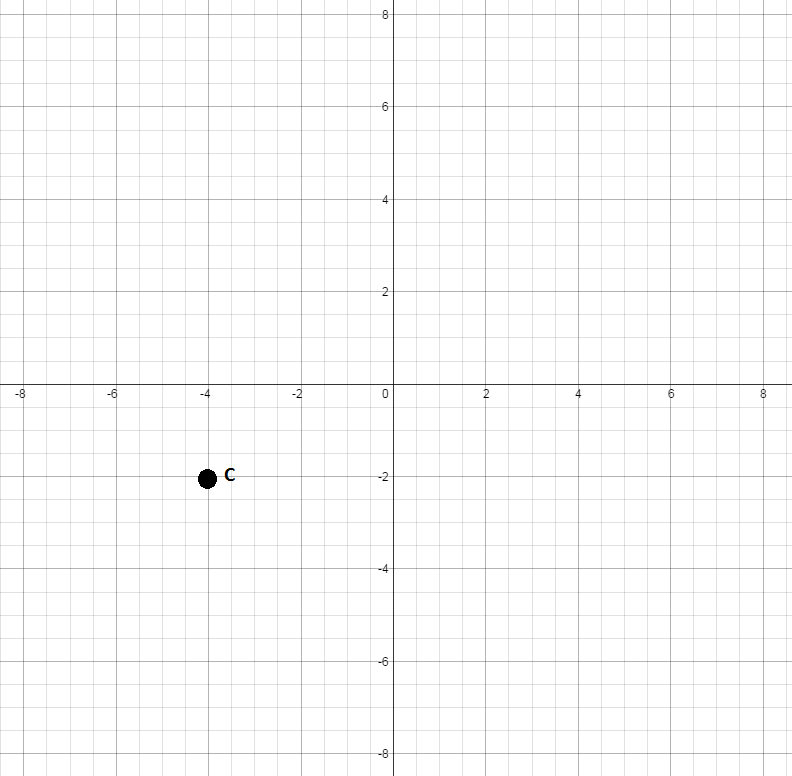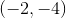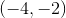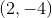Explanation:

Find the x-coordinate by going left on the horizontal x-axis until you come across the line that is directly above the point. The x-coordinate is.

Now, continue down until you reach the point and look across to the vertical y-axis. The y-coordinate is.are the coordinates for point C.

### Example Question #4 : How To Graph A Point

What are the coordinates for point D?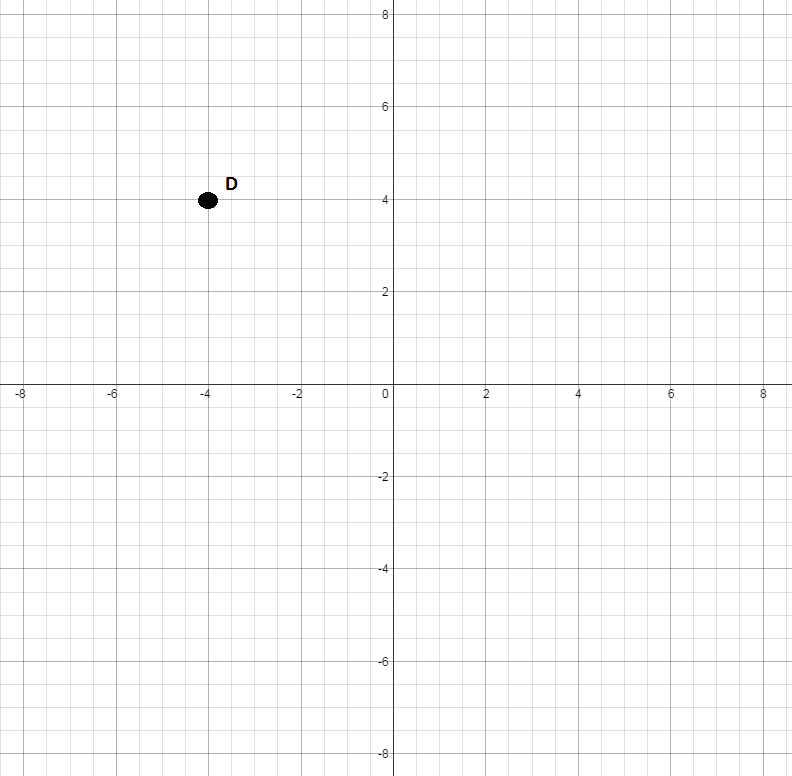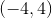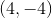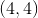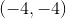Explanation:

Find the x-coordinate by going left on the horizontal x-axis until you come across the line that is directly under the point. The x-coordinate is.

Now, continue up until you reach the point and look across to the vertical y-axis. The y-coordinate is.are the coordinates for point D.

### Example Question #5 : How To Graph A Point

What are the coordinates for point E?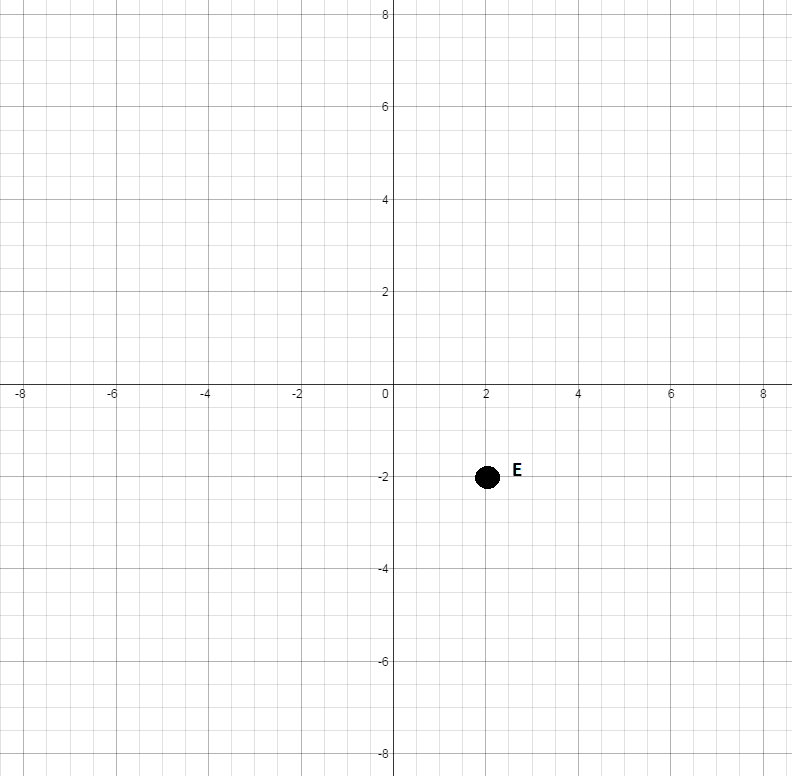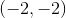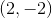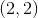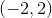Explanation:

Find the x-coordinate by going right on the horizontal x-axis until you come across the line that is directly above the point. The x-coordinate is.

Now, continue down until you reach the point and look across to the vertical y-axis. The y-coordinate is.are the coordinates for point E.

### Example Question #6 : How To Graph A Point

What are the coordinates for point F?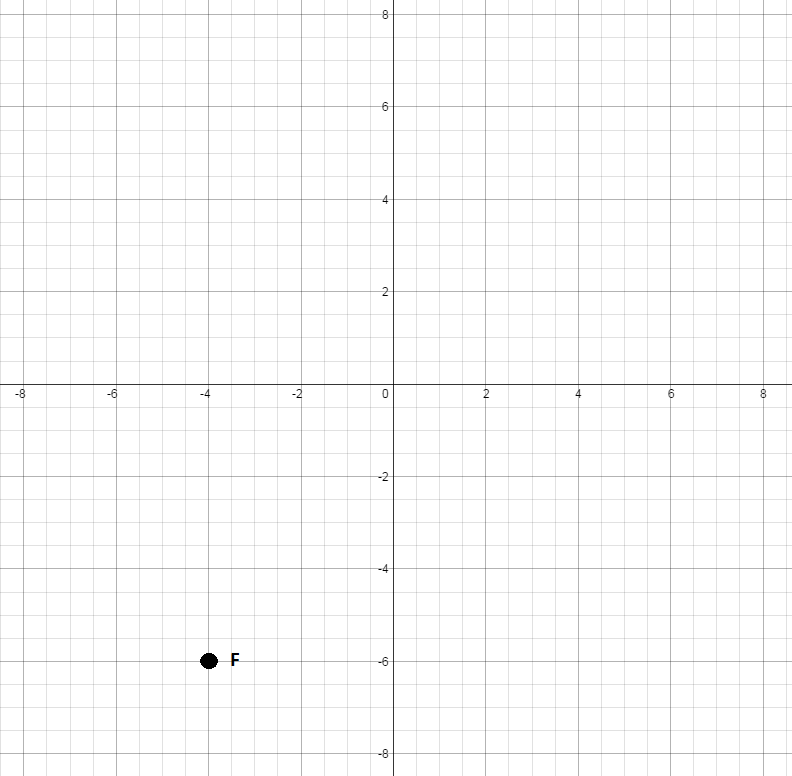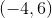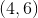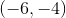Explanation:

Find the x-coordinate by going left on the horizontal x-axis until you come across the line that is directly above the point. The x-coordinate is.

Now, continue down until you reach the point and look across to the vertical y-axis. The y-coordinate is.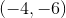are the coordinates for point F.

### Example Question #7 : How To Graph A Point

What are the coordinates for point G?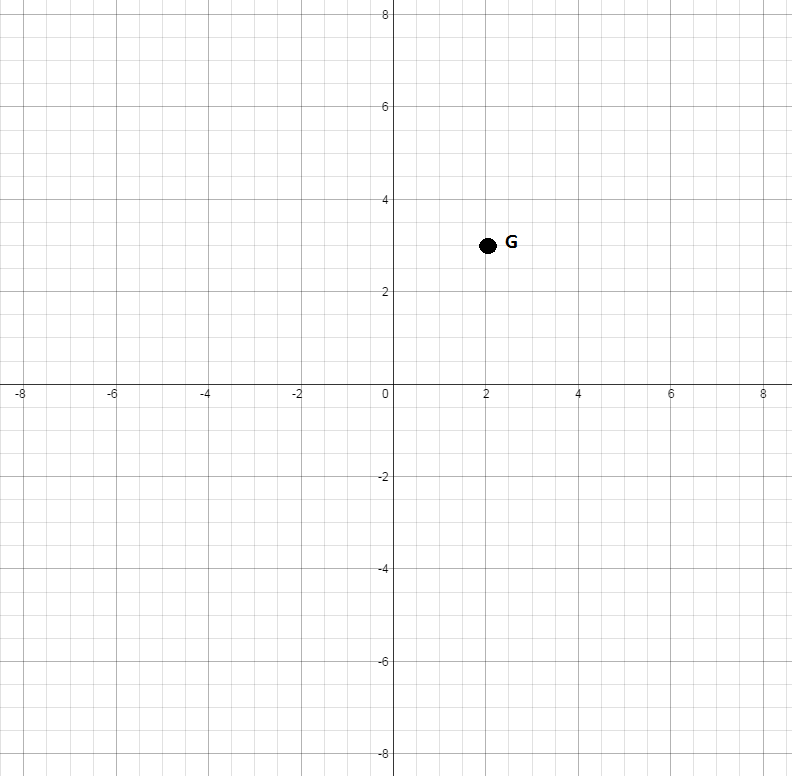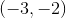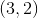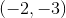Find the x-coordinate by going right on the horizontal x-axis until you come across the line that is directly under the point. The x-coordinate is.
Now, continue up until you reach the point and look across to the vertical y-axis. The y-coordinate is.are the coordinates for point G.